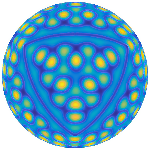Community Profile# Anton Semechko

Last seen: 3 giorni ago Active since 2012

Physiological signal processing, medical and microscopy image processing, computational anatomy, machine learning

#### Statistics

All
••••••••••••#### Content Feed

View by

Submitted

Detect outliers in multivariate datasets
Compute robust estimates of mean and covariance, and flag potential multivariate outliersSubmitted

IsoCut
Extract/visualize level set(s) of a scalar field defined at the vertices of a surface meshSubmitted

Particle tracking on a sphere
Fast particle tracking and ray-triangle intersection queries on triangular meshes of a unit sphereSubmitted

Fast fuzzy c-means image segmentation
Segment N-dimensional grayscale images into c classes using efficient c-means or fuzzy c-means clustering algorithmSubmitted

Exact histogram equalization and specification
Perform exact histogram specification or equalization of 2D grayscale imagesSubmitted

DecimatePoly
Simplify a 2D closed contour/polygon to within user-defined boundary offset toleranceSubmitted

Exact minimum bounding spheres and circles
Compute exact and approximate minimum bounding spheres/circles of 3D/2D point setsSubmitted

Suite of functions to perform uniform sampling of a sphere
Toolbox for generating uniform sampling patterns and decompositions of a unit sphereSubmitted

Rigid body parameters of closed surface meshes
Fast computation of exact rigid body parameters of closed triangular surface meshes using divergence theoremQuestion

none of the axes interactions are working in axes embedded in a UIFigure panel
Problem: The interactions of an axes object embeded in a UIFigure panel are not working. The interactions I am trying to add to ...

6 mesi ago | 0 answers | 0

### 0

PCA on high dimensional data
A 13952736-by-104 data matrix (with observations along rows and variables along columns) will take up 13952736*104*8/2^30 =...

oltre 3 anni ago | 0

| accepted

Fitting scattered data to multiple cosine functions
Below is an example where I use brute-force search to find an optimal set of sinusoid parameters that best fit an unorganized da...

oltre 3 anni ago | 2

| accepted

imshow() not working
Use syntax: imshow(im,[]) when im has non-integer format. Or just do this: im = imread('/MATLAB Drive/My Project/ima...

oltre 3 anni ago | 1

| accepted

distance of points to points calculation
Use <https://www.mathworks.com/help/stats/knnsearch.html#namevaluepairarguments 'knnsearch' function>. For example: [idxA,D_...

oltre 3 anni ago | 0

| accepted

How is the number of bins chosen with the auto binning algorithm in histcounts?
'histcounts' first estimates width of the histogram bins using 'scottsrule': rawBinWidth = 3.5*std(data)/(numel(data)^(1/3...

oltre 3 anni ago | 1

| accepted

Barycentric Coordinates of a spherical triangle
This can be done in four steps: 1) Compute linear transformation (T) that maps triangle A to its counterpart B on the sphere....

oltre 3 anni ago | 0

How do I use a .lut file (or the equivalent in MATLAB) to reference pixels in a grayscale image and assign them new values for pseudocoloring based on the LUT?
There is a Matlab function that maps gray values to RGB values using custom made look-up-tables. Its called 'ind2rgb': <https://...

oltre 3 anni ago | 0

| accepted

Transformation of the complex plan: Conformal Mapping
Modified code ('conformal_map_demo') is attached below. In principle, this piece of code should should allow you to visualize an...

oltre 3 anni ago | 1

How to sum each column element of one matrix with all column elements of another matrix?
Do kron([a b;c d],[1;1]) + kron([1;1],[i j;k l]) Here is an example using symbolic expressions syms a b c d i j k l...

oltre 3 anni ago | 0

| accepted

Generating sets of non repeating random numbers
Here is an example: S=reshape(randperm(40),[],4); All elements in S will be in the range [1 40] and unique. From this it...

oltre 3 anni ago | 1

3D plane plotting restricted by 3 points
Equation of a plane is determined uniquely by any 3 (noncollinear) points lying on the plane. Based on your question, it sounds ...

oltre 3 anni ago | 0

| accepted

Could anyone help me with extracting similar features( to be used as corresponding points)in two images which need to be registered together?

oltre 3 anni ago | 0

| accepted

How can I extract the slices of a 3D matrix in x-direction from a 3D matrix?
Suppose you have G, which is a Y-by-X-by-Z 3D array, then i-th xy slice: G_yx=G(:,:,i); % Y-by-X array i-th xz slice...

oltre 3 anni ago | 2

| accepted

Multivariate nonlinear regression model fitting
If the function you are trying to fit is linear in terms of model parameters, you can estimate these parameters using linear lea...

oltre 3 anni ago | 1

| accepted

How to export 3D spheres (3D plot) generated in Matlab to ANSYS or Abaqus. ?
The builtin 'ellipsoid' and 'sphere' functions produce bad quality meshes (in terms of element shapes and connectivity of the ve...

oltre 3 anni ago | 1

Is there a way to open music and play it?
Yeah, there is a way. Here is an example: % Get sample audio file from the web [y,Fs]=webread('http://www.worldnationala...

oltre 3 anni ago | 1

| accepted

How do I compute the maxpool of a image? Let us say stride of 2,2 on a mxn matrix?
Here is an example: % Sample image im=imread('cameraman.tif'); % sample image % 4 pixels comprising non-overlappi...

oltre 3 anni ago | 0

How do I plot a plane that has points of different values?
Actually, based on the sample picture you provided, you have a total of 17x21 grid points, and total number of cells in the g...

oltre 3 anni ago | 2

| accepted

Obtain Number of Pixels in Area Around Binary Image Object
To distinguish between points/pixels inside the ellipse vs. those outside, you need to know parameters of the ellipse. Here is a...

oltre 3 anni ago | 0

| accepted